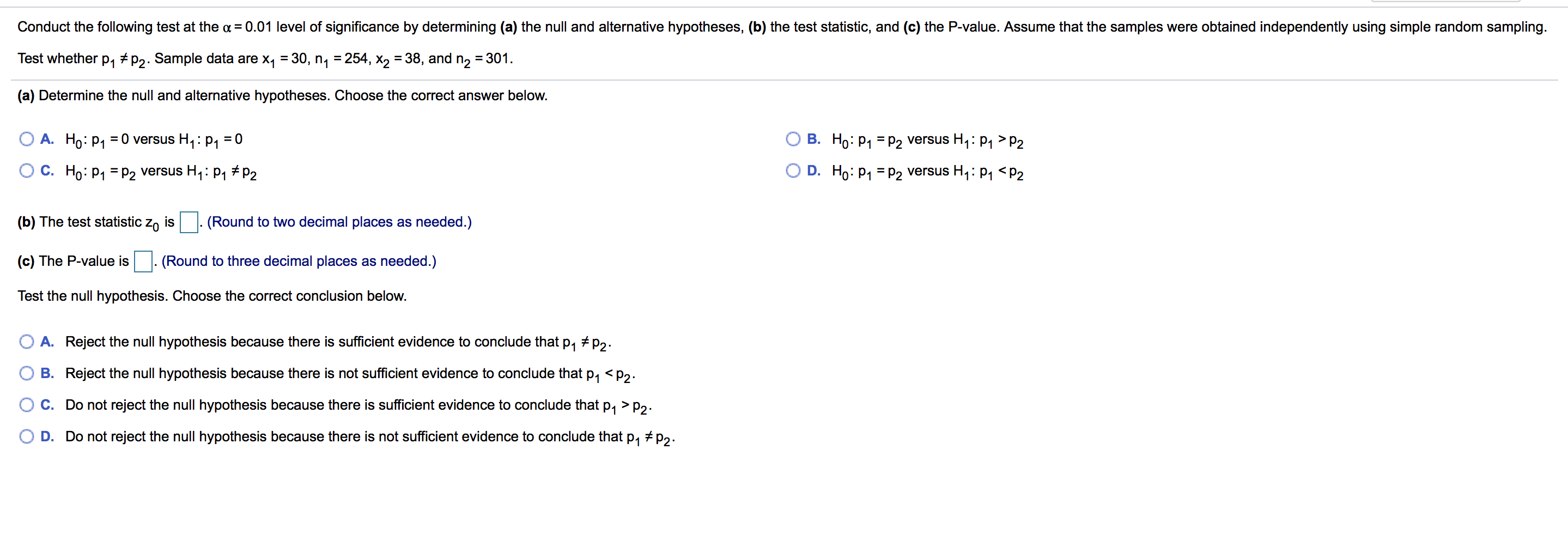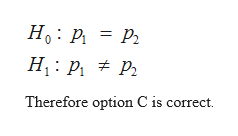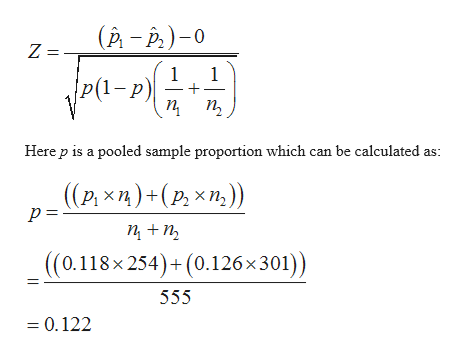# Conduct the following test at the a 0.01 level of significance by determining (a) the null and alternative hypotheses, (b) the test statistic, and (c) the P-value. Assume that the samples were obtained independently using simple random sampling.Test whether p1 #p2. Sample data are x1 30, n, 254, x2 =38, and n2 = 301(a) Determine the null and alternative hypotheses. Choose the correct answer belowA. Ho: p1 0 versus H1: p = 0B. Ho: P1 p2 versus H1: p1 >P2D. Ho: p1 P2 versus H4: p1 P2C. Do not reject the null hypothesis because there is sufficient evidence to conclude thatP1D. Do not reject the null hypothesis because there is not sufficient evidence to conclude that p p2

Questionhelp_outlineImage TranscriptioncloseConduct the following test at the a 0.01 level of significance by determining (a) the null and alternative hypotheses, (b) the test statistic, and (c) the P-value. Assume that the samples were obtained independently using simple random sampling. Test whether p1 #p2. Sample data are x1 30, n, 254, x2 =38, and n2 = 301 (a) Determine the null and alternative hypotheses. Choose the correct answer below A. Ho: p1 0 versus H1: p = 0 B. Ho: P1 p2 versus H1: p1 >P2 D. Ho: p1 P2 versus H4: p1 P2 C. Do not reject the null hypothesis because there is sufficient evidence to conclude that P1 D. Do not reject the null hypothesis because there is not sufficient evidence to conclude that p p2 fullscreen
check_circle

Step 1

The provided information is as follows:

Step 2

a) Null hypothesis state that there is no significant difference between two specified populations.

Alternative hypothesis state that there is no significant difference between two specified populations.

In this case, in order to test the p1≠p2, the hypothesis is defined as:help_outlineImage TranscriptioncloseНо : р. 3 р. Н: р. # Р. Therefore option C is correct fullscreen
Step 3

b) Consider  and  are two sample proportion. The z score t...help_outlineImage Transcriptionclose(р - Р.) -0 Z = 1 1 1-p) п п, Here p is a pooled sample proportion which can be calculated as: ((Р. хп)+(р, хт)) п +п, ((0.118x254)+(0.126x301)) 555 = 0.122 fullscreen

### Want to see the full answer?

See Solution

#### Want to see this answer and more?

Solutions are written by subject experts who are available 24/7. Questions are typically answered within 1 hour.*

See Solution
*Response times may vary by subject and question.
Tagged in

### Other